Smartick is a fun way to learn math!Jan15

# What is an Obtuse Angle?

In today’s post, we are going to learn about what an obtuse angle is with different examples taken from everyday life and some Smartick exercises.

Index

## What is an Obtuse Angle?

An angle is a space shared by two rays that are joined at the same vertex. We can find different types of angles according to their amplitude.

If the amplitude is larger than 90º (90 degrees) and less than 180º (180 degrees) that tells us that it is an obtuse angle.

An obtuse angle is also defined as an angle that is formed when a ray rotates between 90º and 180º around a point.

## Properties of an Obtuse Angle

• These angles measure between 90º and 180º.
• An obtuse angle is larger than a right angle (measures 90º) and an acute angle (measures less than 90º), but it is smaller than a straight angle (180º). In other words, if we order the angles from least to greatest measurement it would be acute< right< obtuse< straight.
• Visually, this angle is more than a quarter of a circle but less than half of a circle. So, if we divide a circle into four parts, an obtuse angle will always occupy between 1/4 and 1/2 of a circle.
• There is always an obtuse angle within an obtuse triangle.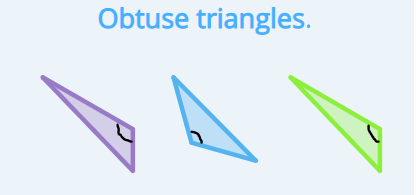## Examples of Obtuse Angles

The following examples show that these angles always measure between 90º and 180º.

• 120º angle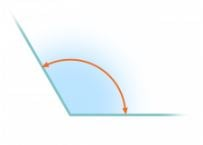This is an obtuse angle because 120 is a number greater than 90 and less than 180. So this angle measurement is between 90º  and 180º: 90º < 120º < 180º.

• 135º angle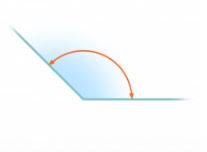This angle is obtuse because 135 is greater than 90 and less than 180, therefore it is between 90º and 180º:  90º < 135º < 180º.

• 160º angle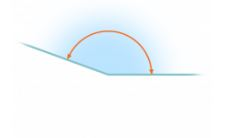This angle is obtuse as well because 160 is greater than 90 and less than 180. It is between 90º and 180º:  90º < 160º < 180º.

## Examples of Obtuse Angles in Everyday Life

Have you ever noticed the obtuse angles that you can find around you? There are quite a few examples.

• Take a look at the hands on this clock. The angle formed by the clock hands is obtuse because it measures between 90º and 180º. On analog clocks, you can find even more examples of times when obtuse angles are formed by the clock hands, for example, 3:35. Can you find more examples? Tell us in the comments!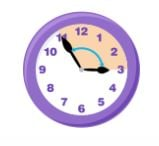• This ballerina is able to stretch her legs to an amplitude that is characteristic of an obtuse angle. If she is able to maintain her balance, and the amplitude, she can turn to the other side and the angle would still measure the same.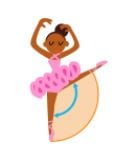• Someone has eaten more than a quarter of this pizza, but less than half. The part of the pizza that is missing is an obtuse angle.• This fan is completely open and forms an obtuse angle. Look closely, if it is closed a little bit, it is a right angle, and if it is closed a bit more but not completely, it is an acute angle.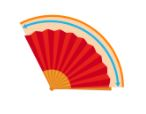• The angle formed by the excavator’s arm forms an obtuse angle so that it will be able to dig into the soil farther away than if it were a smaller angle.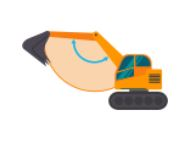## Practice with Obtuse Angles

Now it’s your turn! Read through these Smartick exercises about obtuse angles and try to solve them. After, you can check and see if you have solved them correctly by looking at the answers below.

### Exercise 1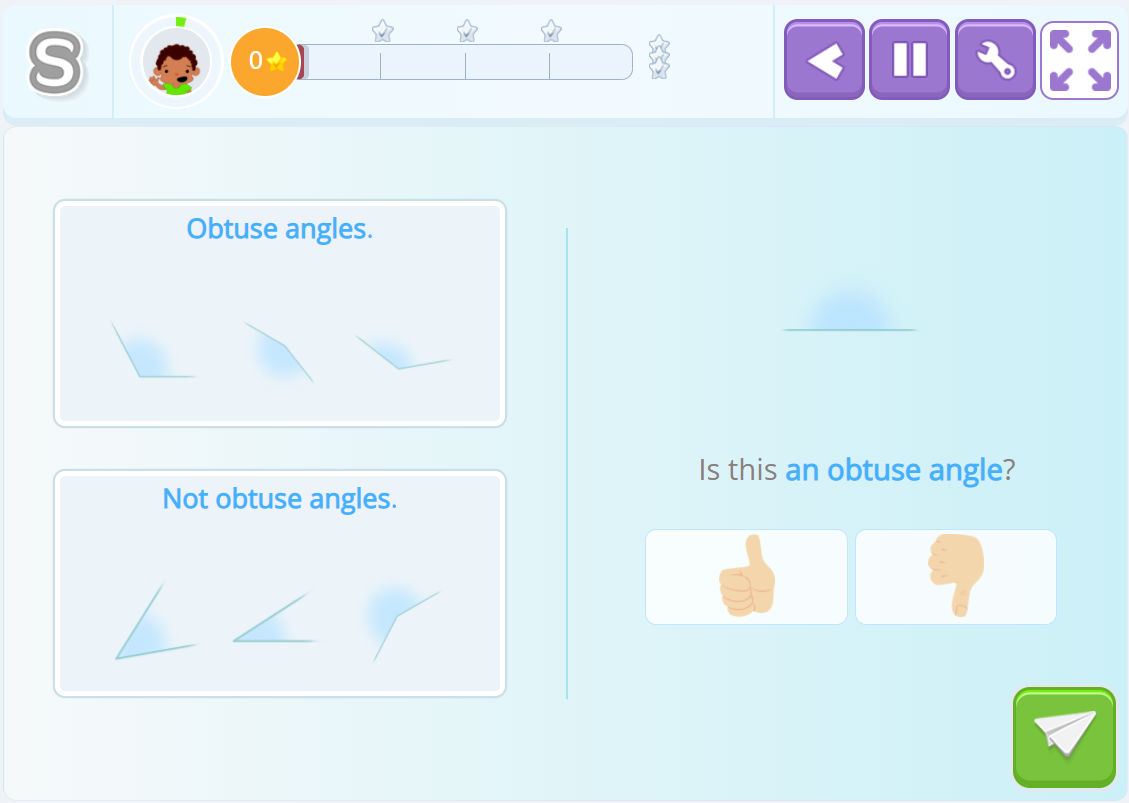### Exercise 2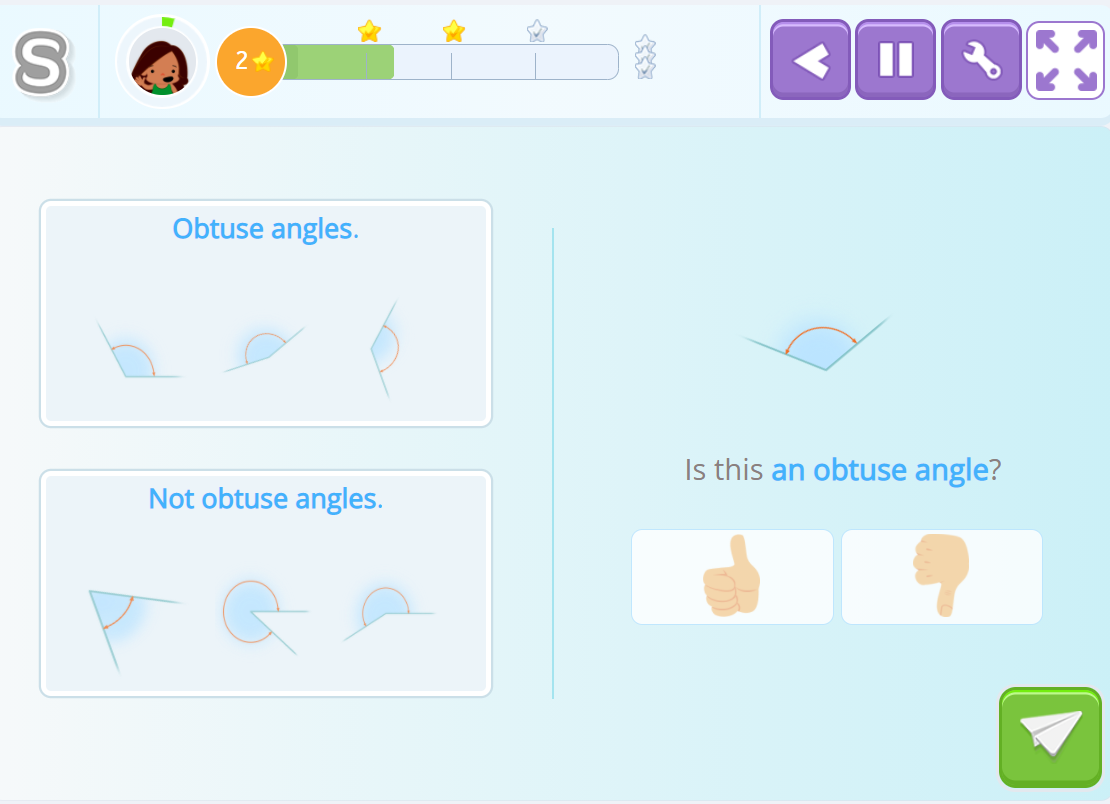### Exercise 3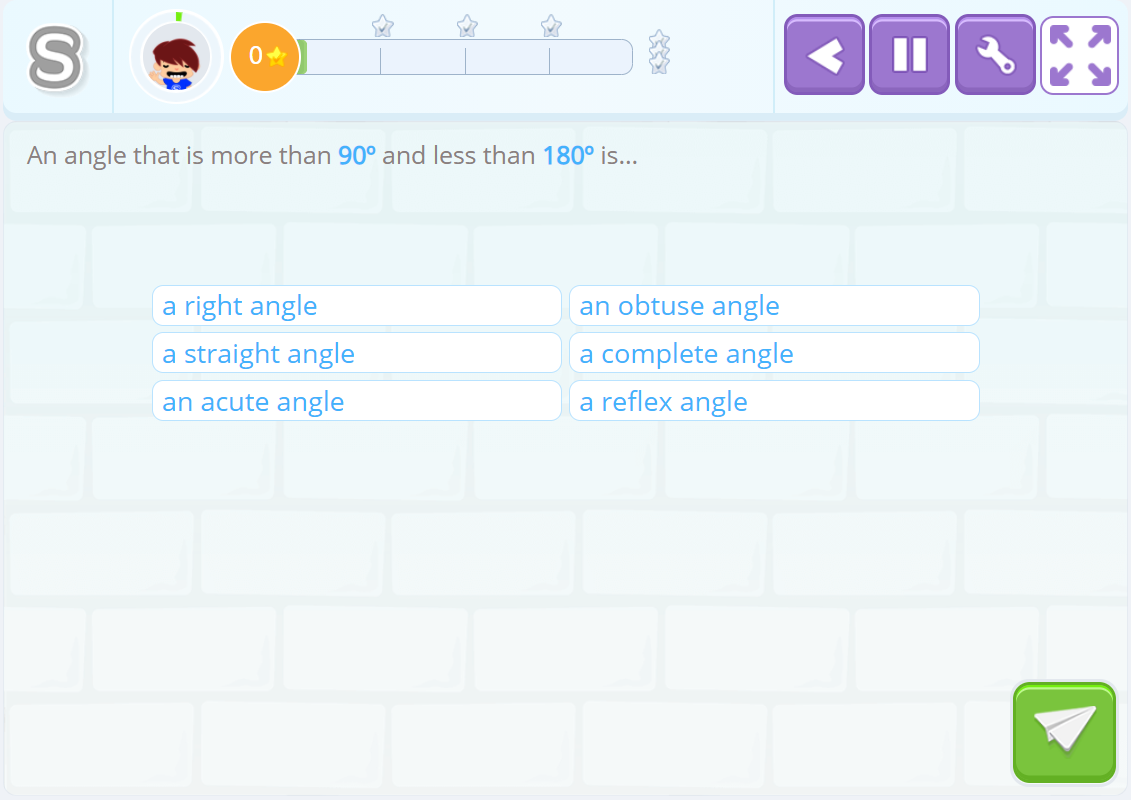### Solutions

• Exercise 1: Yes.
• Exercise 2: No.
• Exercise 3: Obtuse.